Next: Scattering Theory Up: Time-Dependent Perturbation Theory Previous: Spontaneous Emission

# Exercises

1. Demonstrate that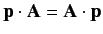when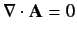, where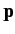is the momentum operator, and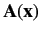is a real function of the position operator,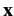. Hence, show that the Hamiltonian (870) is Hermitian.

2. Find the selection rules for the matrix elements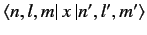,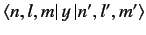, and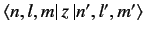to be non-zero. Here,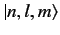denotes an energy eigenket of a hydrogen-like atom corresponding to the conventional quantum numbers,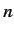,, and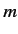.

3. Demonstrate that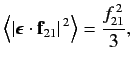where the average is taken over all directions of the incident radiation.

4. Demonstrate that the spontaneous decay rate (via an electric dipole transition) from any 2p state to a 1s state of a hydrogen atom is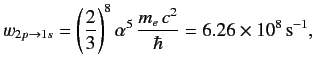whereis the fine structure constant. Hence, deduce that the natural line width of the associated spectral line is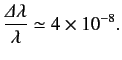The only non-zero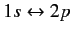electric dipole matrix elements take the values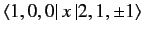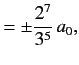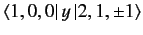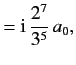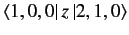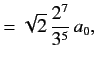where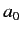is the Bohr radius.Next: Scattering Theory Up: Time-Dependent Perturbation Theory Previous: Spontaneous Emission
Richard Fitzpatrick 2013-04-08